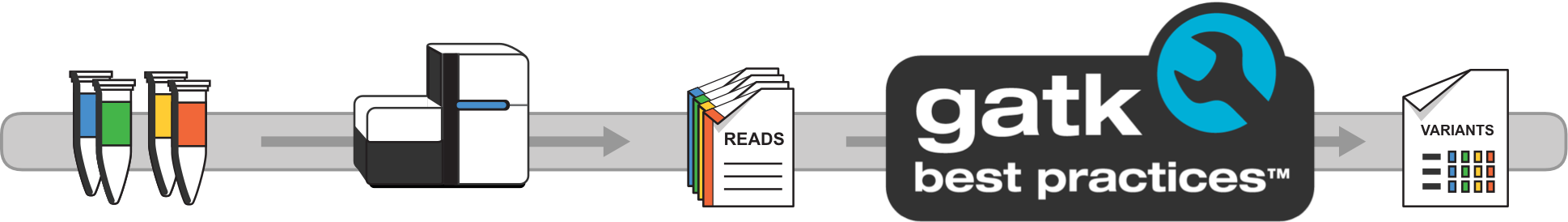# Genome Analysis Toolkit

Variant Discovery in High-Throughput Sequencing Data# Need Help?

Search our documentation

# Community Forum

Hi, How can we help?

Developed in the Data Sciences Platform at the Broad Institute, the toolkit offers a wide variety of tools with a primary focus on variant discovery and genotyping. Its powerful processing engine and high-performance computing features make it capable of taking on projects of any size. Learn more

•Hi Naomie,

Here is an article on how active regions are calculated in HaplotypeCaller. Hopefully, this will answer your question.

Kind regards,

Pamela

•Hi Pamela,
thank you for your quick answer. I still have trouble understanding how the per score probability is computed: it is explained that the reference confidence model is used, but in this other post on reference confidence model (https://gatk.broadinstitute.org/hc/en-us/articles/360035531532-HaplotypeCaller-Reference-Confidence-Model-GVCF-mode-) I don't find an explanation of the exact formula needed to compute these probabilities, do you use bayes' theorem to compute the following point? :
"Estimate the confidence that no indel of size X (determined by command line parameter) could exist at this site by calculating the number of reads that provide evidence against such an indel, and from this value estimate the chance that we would not have seen the allele confidently"
(especially the last part of the sentence) and if not then how to you compute it?

thank you,
Naomie.

•HaplotypeCaller does use Bayes' Theorem to calculate the genotype probability. This article includes a breakdown of the specific formulas that are used to calculate these probabilities:

Kind regards,

Pamela

•Hi Pamela,

actually I was talking about the score probability computed to define whether a region is active or not. The article that you mentioned in your first answer (ActiveRegion determination (HaplotypeCaller and Mutect2) – GATK (broadinstitute.org)) explains that:

1. for each locus an activity score is calculated and

2. then from that, the raw activity profile is smoothed to get the actual activity profile

3. and finally comparing it to a given threshold allows to set a region as active.

About step 1 it is written: "per-position score is the probability that the position contains a variant as calculated using the reference-confidence model applied to the original alignment"

My question is what is the formula to calculate this above probability ? (i.e. what does reference confidence model means in this case?)

Thank you,

Naomie.

•I have been looking into this and trying to find some information on the algorithms behind active region determination. I was able to find this resource with a section specifically about active region determination on page 12: https://www.biorxiv.org/content/10.1101/201178v3.full.pdf

•